How-Tos

# Advanced Transformation and Formulas Part 2This is a continuation of a ProductHour that introduced the power of transforming data in Workato using Formulas. You can check it out here.

### Introduction

Transforming data is not always as straightforward as manipulating the format of a single field. Often, it involves a collection of records to transform; as opposed to working with a single record. Also, many use cases require a combination of formulas to achieve the transformation you want.

### Making formulas work together

Formulas are powerful tools with which you can transform the raw data into results your business processes needs. However, the power of using formulas truly manifests when they work together. Formulas can be chained together to transform your data exactly how you need it. A very typical challenge faced in integration is the need to deal with a one-to-many (parent-child) relationship.

Take the example of an invoice with multiple line items. At the top, there is information about the customer, date and payment mode. At the same time, there are multiple line items in the invoice, each with information about the item, amount and quantity. When you want to extract and transform specific values from this collection of items, formulas can be very useful.

#### Summarizing a collection of data

The most common use case we see involves syncing financial objects like invoices. These records are typically a parent record with many child records (line items). This can get tricky when users try to sync summarized information from one application to another. When building such a recipe, you may wonder: “I have the entire collection of items, but I don’t need all the information in it. Actually, I only want to grab all the values from a specific field (say the amount in each line item). How can I get the total amount from each line item?” This is where Array helper formulas are extremely useful! Let’s take a look at one of my favourites, pluck.

#### `pluck`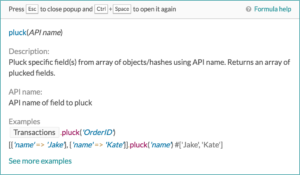True to its name, this formula plucks specific fields from an array of objects and returns an array of these values. With this, we can very easily extract the most important piece of information from an Array of objects without worrying about the size of the Array or looping through the Array and collecting these values in a temporary variable for use elsewhere. Now, how can we use this in our integration use cases?

#### Use case 1: Sum invoice total amount to a field in Salesforce

Harry is an integration expert in the finance department and is in charge of syncing all invoice information into Salesforce. Besides making sure the invoice is created, the requirements involve mapping the sum of line item amounts to a field in Salesforce. A raw invoice record will be in this form.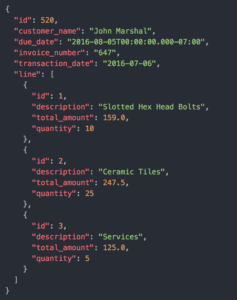Harry needs to extract the total_amount values from each line and add them up. The formula that he used to achieve this is really elegant and perfect for this use case. Let’s take a look.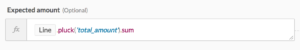First, he plucks the amount value in the invoice line items. This returns an Array of numbers that will look like this: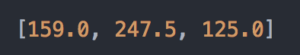Next, we chain the formula sum  to add these values and arrive at a single total amount.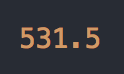#### join

Now, what if we’re not working with numbers that can be easily summed? Alphanumeric values cannot be summed, but can be joined into a single string.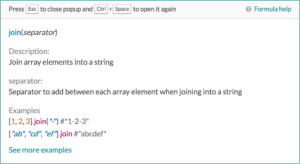This formula takes an array of values and joins each item, using a user-defined separator, into a single string.

#### Use case 2: Summarize line item details into a single field

Harry has another set of requirements. He needs to extract a list of all item descriptions in the invoice and summarise them into a note field. Instead of adding up the values, Harry needs to use the join formula to join a list of strings.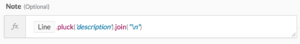OR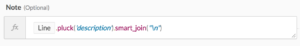Once again, Harry makes use of `pluck` to extract the description from each line item. This results in an array of string values of each item description.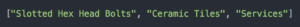Next, he use either one of the 2 join formulas to form a single string. Here, the newline control character (`\n`) is used to join the titles. This means that the message will contain one title per line to improve readability.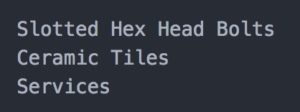#### `join` vs `smart_join`

Why are there 2 types of join formula? Why is one smarter than the other? Looking at the description of `smart_join`, we can see an important difference.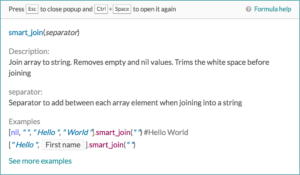`smart_join` removes empty values and trims any peripheral white spaces before joining the values. Ok – but how is this useful? To understand how, we need to understand that data returned from triggers or actions may not always be consistent. Sometimes, we may get fields that are empty. In these scenarios, we may want to drop them instead of having empty values in our final string.

#### Filtering from a Collection of Data

What if Harry needs to do some validation before summarizing the line items to the note field. It is very common for businesses to only include inventory items and exclude services from these summary. Workato created a custom formula where for this very reason.

#### `where`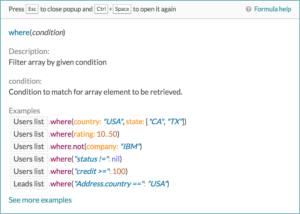We can use this formula to filter an array of objects by a given condition we specify. We can then apply this on a single field or across multiple fields. There are also multiple operators (equals, more than, less than or equal to, etc.) that we can use to compare values in each object.

#### Use case 3: Selecting inventory line items

Harry now needs to select only inventory items from the invoice line items list. This can be achieve by including a where formula before the `pluck` and join.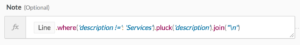where conditions to filter line items

In this example, the line items are first filtered down to a subset of the initial list of line items using the `where` formula. Because of the `where` condition, this subset does not contain any items with Services as it’s description value.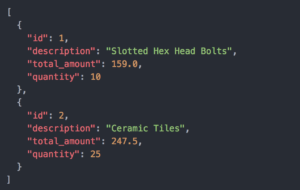At this point, the same `pluck` and `join` formula chain is applied to transform the array in a summarized note with only inventory items.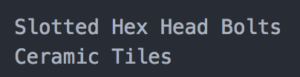### Endless possibilities

That wasn’t so hard, was it? If you’re looking for more ways to transform your data to suit your needs, head over to the Workato Formula documentation for a full list of formulas.

Previous Post

Next article